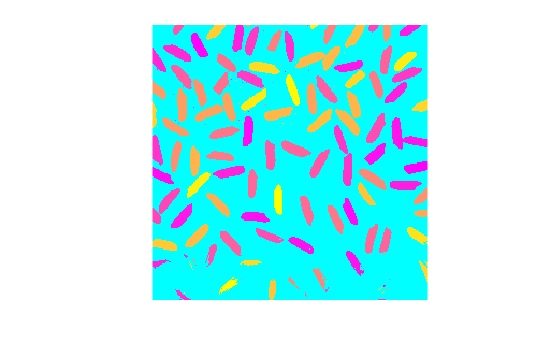# label2rgb

Convert label matrix into RGB image

## Syntax

``RGB = label2rgb(L)``
``RGB = label2rgb(L,cmap)``
``RGB = label2rgb(L,cmap,zerocolor)``
``RGB = label2rgb(L,cmap,zerocolor,order)``

## Description

example

````RGB = label2rgb(L)` converts a label matrix, `L`, such as those returned by `labelmatrix`, `bwlabel`, `bwlabeln`, or `watershed`, into an RGB color image for the purpose of visualizing the labeled regions. The `label2rgb` function determines the color to assign to each object based on the number of objects in the label matrix. The `label2rgb` function picks colors from the entire range of the color map.```
````RGB = label2rgb(L,cmap)` specifies the colormap `cmap` to be used in the RGB image.```
````RGB = label2rgb(L,cmap,zerocolor)` specifies the RGB color of the background elements (pixels labeled `0`).```
````RGB = label2rgb(L,cmap,zerocolor,order)` controls how `label2rgb` assigns colors to regions in the label matrix.```

## Examples

collapse all

Read an image and display it.

```I = imread('rice.png'); imshow(I)```Create a label matrix from the image.

```BW = imbinarize(I); CC = bwconncomp(BW); L = labelmatrix(CC);```

Convert the label matrix into RGB image, using default settings.

```RGB = label2rgb(L); figure imshow(RGB)```Convert the label matrix into an RGB image, specifying optional parameters. This example uses the `'spring'` colormap, sets background pixels to the color cyan, and randomizes how colors are assigned to the labels.

```RGB2 = label2rgb(L,'spring','c','shuffle'); figure imshow(RGB2)```## Input Arguments

collapse all

Label matrix of contiguous regions, specified as an m-by-n matrix of nonnegative integers. Pixels labeled `0` are the background. Pixels labeled `1` make up one object; pixels labeled `2` make up a second object; and so on.

Data Types: `single` | `double` | `int8` | `int16` | `int32` | `int64` | `uint8` | `uint16` | `uint32` | `uint64` | `logical`

Color map, specified as one of the following.

Value

Description
c-by-3 matrix of data type `double`

Color map matrix specifying c colors, each as an RGB triple. c must be greater than or equal to the number of labels, numlabels, in label matrix `L`. You can compute the number of labels as ```numlabels = max(L(:))```.

If c is greater than numlabels, then `label2rgb` creates the `RGB` image using only the first numlabels rows in the matrix.

colormap function

Name of a MATLAB® colormap function, such as `'jet'` or `'gray'`. See `colormap` for a list of supported colormaps.

colormap handle

Handle of a colormap function, such as `@jet` or `@gray`.

Label matrix of contiguous regions, specified as a 3-element vector representing an RGB triple, or one of the following color abbreviations.

Value

Color

`'b'`Blue
`'c'`Cyan
`'g' `Green
`'k'`Black
`'m'`Magenta
`'r'`Red
`'w'`White
`'y'`Yellow

Color order, specified as `'noshuffle'` or `'shuffle'`. The `'noshuffle'` order arranges colormap colors to label matrix regions in numerical order. The `'shuffle'` order assigns colormap colors pseudorandomly.

## Output Arguments

collapse all

RGB image, returned as an m-by-n-by-3 numeric matrix.

Data Types: `uint8`